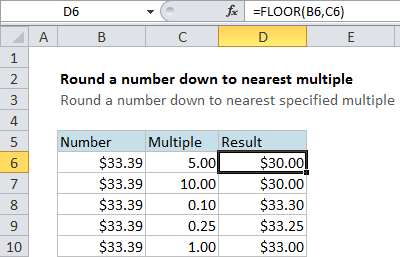## Excel Office

Excel How Tos, Tutorials, Tips & Tricks, Shortcuts

# Round a number down to nearest multiple in Excel

This tutorials shows how to Round a number down to nearest multiple in Excel.

If you need to round a number down to the nearest specified multiple (i.e. round a number down to the nearest dollar, down to the nearest \$.25, down to the nearest multiple of 5, etc.) you can use the FLOOR function.

Worked Example:   MROUND function: Description, Usage, Syntax, Examples and Explanation

## Formula

`=FLOOR(number,multiple)`## Explanation

In the example, the formula in cell D6 is

`=FLOOR(B6,C6)`

This tells Excel to take the value in B6 (\$33.39 ) and round it down to the nearest multiple of the value in C6 (5). The result is \$30.00, since 30 is the multiple of 5 before 33.39. Likewise, in cell D7, we get 30 when rounding down using a multiple of 10.

Worked Example:   CEILING function: Description, Usage, Syntax, Examples and Explanation

You can use FLOOR to round prices, times, instrument readings or any other numeric value.

Worked Example:   Round to nearest 5 in Excel

Note that FLOOR rounds down using the multiple supplied. You can use the MROUND function to round to the nearest multiple and the CEILING function to round up with a multiple.